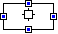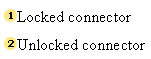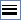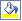# Lab 10: Simple programming in Excel and Measuring Uncertainty

### Contents

• Objectives
• To have completed all previous labs.
• To become more comfortable with Microsoft Excel.
• To understand simple programming concepts using Excel.
• Develop another algorithm to identify students with grades above the average.
• Calculate the probability of each letter grade
• Calculate the Shannon entropy of the letter grade probability distribution.
• Compare the Shannon entropies of three professors: Matic, Churchill and Mussolini
• Question 1: In which professor's class (Matic, Chruchil, and Mussolini) is the final grade more uncertain? Which one is more certain?
• Question 2: Does the Shannon entropy help in deciding which of the three courses to take? How?
• Create a flowchart for the first algorithm, post flowchart on your blog. Also comment on your blog what is the difference between the Hartley and Shannon measures of information and how do they measure information.
• Deliverables
• An Excel file called lab10.xls containing :
• An algorithm to transform percentage scores into letter grades.
• An algorithm to mark the students with grades above the average.
• Calculations for probability of each letter
• Calculations of the Shannon entropy of letter grades.
• Flowchart for the first algorithm, posted on your blog. Also comment on your blog what is the difference between the Hartley and Shannon measures of information and how do they measure information.
• Comparisons of entropy for three professors: Matic, Churchill and Mussolini.
• Answer questions 1 and 2
• Completion Time

• Acknowledgements:

## Introduction

Professor Matic is a new IUB faculty who not familiar with the letter grading system. He does not know how to translate grades from percentages into letters. The School of Informatics is hiring I101 students to develop the ultimate application to transform percentage score into letter grades.

You need to edit an Excel spreadsheet (i) with an algorithm to transform percentage scores into letter grades and (ii) create another algorithm to identify the students with grades above the average. You also need to (iii) Calculate the probability distribution for each letter grade and (iv) Produce a flowchart for algorithm (i) and publish it in your Blog.

Finally, you need to (v) compare the Shannon entropies of three professors: Matic, Churchill and Mussolini. You will find information about these professors on the second book of the spreadsheet. The grade probability distribution for the three professors is provided there. You should briefly comment your results on the spreadsheet and (vi) answer the following two questions: Question 1: In which professor's class (Matic, Chruchil, and Mussolini) is the final grade more uncertain? Which one is more certain?, Question 2: Does the Shannon entropy help in deciding which of the three courses to take? How?

NOTE: The excel spreadsheet provided has two books, you'll need them both

# the if statement

IF

Returns one value if a condition you specify evaluates to TRUE and another value if it evaluates to FALSE.

Use IF to conduct conditional tests on values and formulas.

Syntax

IF(logical_test,value_if_true,value_if_false)

Logical_test is any value or expression that can be evaluated to TRUE or FALSE. For example, A10=100 is a logical expression; if the value in cell A10 is equal to 100, the expression evaluates to TRUE. Otherwise, the expression evaluates to FALSE.

Value_if_true is the value that is returned if logical_test is TRUE. For example, if this argument is the text string "Within budget" and the logical_test argument evaluates to TRUE, then the IF function displays the text "Within budget". If logical_test is TRUE and value_if_true is blank, this argument returns 0 (zero). To display the word TRUE, use the logical value TRUE for this argument. Value_if_true can be another formula.

Value_if_false is the value that is returned if logical_test is FALSE. For example, if this argument is the text string "Over budget" and the logical_test argument evaluates to FALSE, then the IF function displays the text "Over budget". If logical_test is FALSE and value_if_false is omitted, (that is, after value_if_true, there is no comma), then the logical value FALSE is returned. If logical_test is FALSE and value_if_false is blank (that is, after value_if_true, there is a comma followed by the closing parenthesis), then the value 0 (zero) is returned. Value_if_false can be another formula.

 1 2 3 4
 A Score 45 90 78

 Formula Description (Result) =IF(A2>89,"A",IF(A2>79,"B",IF(A2>69,"C",IF(A2>59,"D","F")))) Assigns a letter grade to the first score (F) =IF(A3>89,"A",IF(A3>79,"B",IF(A3>69,"C",IF(A3>59,"D","F")))) Assigns a letter grade to the second score (A) =IF(A4>89,"A",IF(A4>79,"B",IF(A4>69,"C",IF(A4>59,"D","F")))) Assigns a letter grade to the third score (C)

In the preceding example, the second IF statement is also the value_if_false argument to the first IF statement. Similarly, the third IF statement is the value_if_false argument to the second IF statement. For example, if the first logical_test (Average>89) is TRUE, "A" is returned. If the first logical_test is FALSE, the second IF statement is evaluated, and so on.

The letter grades are assigned to numbers using the following key (in the lab we shall use a different key).

 If Score is Then return Greater than 89 A From 80 to 89 B From 70 to 79 C From 60 to 69 D Less than 60 F

# using log

LOG

Returns the logarithm of a number to the base you specify.

Syntax

LOG ( number ,base)

Number is the positive real number for which you want the logarithm.

Base is the base of the logarithm. If base is omitted, it is assumed to be 10.

Example

 1 2 3 4
 A B Formula Description (Result) =LOG(10) Logarithm of 10 (1) =LOG(8, 2) Logarithm of 8 with base 2 (3) =LOG(86, 2.7182818) Logarithm of 86 with base e (4.454347)

drawing a flowchart

1. On the Drawing toolbar  (toolbar: A bar with buttons and options that you use to carry out commands. To display a toolbar, click Customize on the Tools menu, and then click the Toolbars tab.) , click AutoShapes , point to Flowchart , and then click the shape you want.
2. Click where you want to draw the flowchart shape.
3. If you want to add additional shapes to the flowchart, repeat steps 1 and 2, and then arrange them in the order you want.
4. Add connectors between each of the shapes
• On the Drawing toolbar, click AutoShapes , point to Connectors , and then click the connector line you want.
• Point to where you want to lock the connector. Connection sites appear as blue circles as you pass the pointer over a shape.• Click the first connection site you want, point to the other shape, and then click the second connection site. Locked connectors will keep the shapes connected even when you move the shapes.

•• Add text to the shapes by right-clicking the shape, then click Add text , and start typing.
• Note: You cannot add text to a line or connector; use a text box  (text box: A movable, resizable container for text or graphics. Use text boxes to position several blocks of text on a page or to give text a different orientation from other text in the document.) to place text near or on these drawing objects.
5. Change the line style or add color to the connectors:
• Select the line or connector you want to change.
• Do one of the following :
• On the Drawing toolbar, click the arrow next to Line Color• To change to the default color, click Automatic .
• To change to another color, click one of the colors below Automatic , then change the style of a line or connector
• On the Drawing toolbar, click Line Style•

• Click the style you want; or click More Lines , and then click a style.

6. Add color or fills to the shapes.
• Select the shape you want to change.
• On the Drawing toolbar, click the arrow next to Fill Color• If you want to change colors, click one of the colors below Automatic

If you want to add a gradient, patterned, textured, or picture fill, click Fill Effects, and then click the tab you want and select options

Check that you have completed your deliverables before you leave.